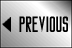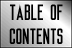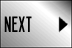STAR FLEET BATTLES ERRATA

## Star Fleet Battles Errata: (H2.4) through (H7.53)

(H2.4) Reference to (C2.11) should be to (C2.111).

(H2.5) The Penal ships are in Module R3 (R3.R5).

(H3.3) Reference (C2.1) should be to (C2.16).

(H3.4) Reference changes: (G7.36-C) should be to (G7.36-B); (P3.4) should be to (P3.434).

(H6.1) Reference to (D22.0) should be to (D22.15); see (H6.5). (H7.111) Reference to (H7.34) should be to (H7.4).

(H7.132) Add to last sentence: This is not the same meaning for "expended" as used in (D22.0).

(H7.133) Reference to (J3.12) should be to (J3.122).

(H7.2) Change to: "Begin arming multi-turn weapons (H7.53) and other systems (H7.32)." Reference to (G24.214) should be to (G24.2214). PPDs cannot receive reserve power while firing.

(H7.31) Reference to (D21.32) should be to (D21.31).

(H7.32) Reference to (J3.12) should be to (J3.122).

(H7.35) ...or braking energy (C3.53).

(H7.36) Should have been a space between this rule and the next higher. Also note that power from the batteries can be sent to phaser capacitors on the same turn that the capacitor is emptied by firing the phasers. This is a particular tactic used by Andromedans to create extra room in their batteries to put power from the panels into.

(H7.42) Reference to (C3.52) should be to (C3.53). Reference to (C6.0) should be to (C6.22). Reference to (C10.0) should be to (C10.113).

(H7.43) EXAMPLE: Change "warp power" to "warp engine power" twice in this example.

(H7.47) Reference to (C10.0) should be to (C10.113).

(H7.48) Reference to (C10.0) should be to (C10.113).

(H7.49) Reserve warp power can be used to execute an HET before allocated power for such an HET is used. If the allocated power is never used, it would simply be lost. An Andromedan ship would treat such allocated power as never generated under (D10.74).